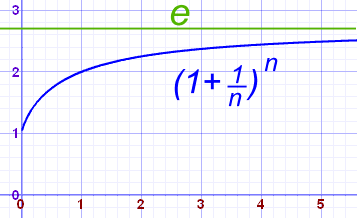# Euler numbers in c++ -- How to

Explanation:
Below mention code is compiled in Visual Studio 2015 and Code Blocks 13.12,output snap is attached.. If any problem you feel and you want some explanation feel free to contact us.

# e (Euler's Number)The number e is a famous irrational number, and is one of the most important numbers in mathematics.
The first few digits are:

2.7182818284590452353602874713527 (and more ...)
It is often called Euler's number after Leonhard Euler.
And Euler is spoken like "Oiler".
e is the base of the Natural Logarithms (invented by John Napier).
e is found in many interesting areas, so it is worth learning about.

## Calculating

There are many ways of calculating the value of e, but none of them ever give an exact answer, because e is irrational (not the ratio of two integers).
But it is known to over 1 trillion digits of accuracy!
For example, the value of (1 + 1/n)n approaches e as n gets bigger and bigger:n (1 + 1/n)n 1 2.00000 2 2.25000 5 2.48832 10 2.59374 100 2.70481 1,000 2.71692 10,000 2.71815 100,000 2.71827

Code:

/**************************************************|
/*************C++ Programs And Projects************|
***************************************************/
#include<iostream>
#include<math.h>
using namespace std;
void main() {
int Number = 0;
cout << "Enter Ending Point for Euler Numbers = ";
cin >> Number;
for (float i = 1; i <= Number; i++) {
//(1 + 1 / n)n
float tempResult = ((1 / i) + 1);
float result = pow(tempResult, i);
cout << i << " == " << result << endl;
}

}

Output: